## Math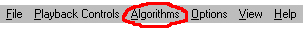FractMus 2000 generates musical material using twelve algorithms from number theory, chaotic dynamics, fractals and cellular automata. This yields the composer an almost inexhaustible source of melodic material. Each voice can use any algorithm independently from the others. You can see a graphical representation of the algorithm by pressing the Visualize button.

• Morse-Thue Sequence
• Earthworm Sequence
• Wolfram one-dimensional cellular automata
• 3n+1 numbers
• Logistic Map
• 1/f Noise
• Henon
• Hopalong
• Martin
• Lorenz
• Random

### Morse-Thue Sequence

Consider the following sequence of the non-negative integers 0, 1, 2, 3, 4, 5... written in binary notation:

0, 1, 10, 11, 100, 101...

take the sum of the digits modulo 2 (known as the "digital root") of each number, thus forming the following sequence:

0, 1, 1, 0, 1, 0, 0...

This is the so-called Morse-Thue Sequence. This sequence can also be generated by iterating the map 0 -> 01 and 1 -> 10. Starting with a single "0" you get:

0 1 1 0 1 0 0 1 1 0 0 1 0 1 1 0...

Also, each generation can be created appending the complement of the preceding one, as follows:

0 1 1 0 1 0 0 1 1 0 0 1 0 1 1 0...

Of course, you are not restricted to base 2. You can use any counting system you like. Furthermore, the sequence shows a high degree of self-similarity; for instance, if every even term in the sequence is removed the sequence remains unchanged! Also, removing every second couple keeps the sequence unchanged.

How do you make music out of this sequence?. Here is how:
First you select a base in which to count (the original sequence is in base 2). Second you choose a number by which the general counters will be multiplied (the multiplier). Now, this is what happens: The number shown in the Start Counter is multiplied by whatever value you have chosen for the multiplier, then the result is converted to the base you have chosen, for example:

base 3 multiplier 3 (counter 17) = 3*17 = 51 -> 3 = 1220.

The digits of this number are then added (in base 10):

1+2+2+0 = 5

and made the modulo operation with the number of notes in the scale  times the number of octaves . Assuming C major (7 notes) and 1 octave, this yields:

5%(7*1) = 5,

which is then is added to the starting note note in MIDI values (middle C is No. 60). Since the mapping starts at 0, then the resulting note is an A above middle C.
Some base-multiplier combinations yield an amazing variety, while others are boringly static. The result depends in the right combination of base, multiplier and scale.

### Earthworm Sequence

"Earthworm" algebra was first discussed by Clifford A. Pickover in his book Computers and the Imagination. While digging in his garden Clifford accidentally severed an earthworm. It is known that both parts of a severed earthworm generally continue growing, so Mr. Pickover did not worry to much about the fate of the earthworm. However, he devised a method of multiply-and-severe which he called "Earthworm algebra". It works as follows: take any whole number A, a constant multiplier B and a number which will be the maximum number of digits allowed, C. Next, multiply A and B. Take the result and multiply it again by B. Repeat this process until the number of digits in the result get past C. Now, severe the result by truncating it to the its rightmost C digits. Multiply again by B and severe again to C digits, and so forth. It turns out that all earthworms (mathematically speaking) eventually enter a cycle, an infinite loop of repeating values. A simple example: lets take 2 as our first number, 3 as the multiplier and 2 for the maximum number of digits. The sequence will go as follows:

6 18 54 62 (severed 162) 86 58 74 22 66 98 94 82 46 38 14 42 26 78 34 2 [6 18...

This particular combination gives a cycle of 20 different values. Some combinations generate "worms" that are thousands of values long. This simple procedure yields great complexity. The generated sequences seem to be almost random, but if you listen to them you'll notice hidden patterns. I believe that aural inspection of these algorithms reveals information that would otherwise go unnoticed.

### Wolfram one-dimensional cellular automata

The mathematician Stephen Wolfram created a one-dimensional variation of von Neumann cellular automata which develops on a one-dimensional universe (a line). In this type of CA, each cell is surrounded by only two other cells, its two immediate neighbors on either side. Each succeeding generation is represented by a line underneath the preceding one. A cell in generation 2 determines its state (dead or alive) by looking at the state of the cell directly above it (that is, in generation 1) and at that cell's two neighbors. There are only eight possible combinations of the states of those 3 cells, ranging from "AAA" (all alive) to "DDD" (all dead). Since there are only eight possible states for the ancestors of any given cell, and these states may result in one of two states for the new cell (dead or alive), there are 256 (2 to the 8th power) possible rulesets for this type of cellular automata. Wolfram classified all 256 rules in four different "classes":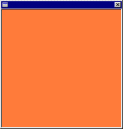Class I are rules that generate boring configurations, such as all dead or all alive cells.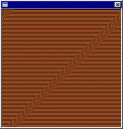Class II includes "frozen" configurations, where all initial activity eventually settles down to stable structures.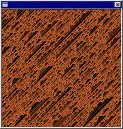Class III rules generate chaotic configurations, resembling noise patterns.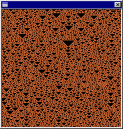Class IV rules display complex but not random behavior. The output is highly sensitive on initial conditions (cell configuration).

These universes are one-dimensional tori, that is, the last cell connects with the first cell. It is remarkable how the aural representation resembles the graphical output of these CA. You can very clearly hear the differences between all four classes and how they match perfectly with their graphical counterpart.

### 3n+1 Numbers

Take any whole number greater than 1. If it is even, divide it by two, if odd, multiply by 3 and add 1; continue with this process until you reach the number 1. This is the procedure to generate 3n+1 number sequences, a.k.a. hailstone numbers. Take for example the number 7. It generates the following sequence:

7, 22, 11, 34, 17, 52, 26, 13, 40, 20, 10, 5, 16, 8, 4, 2, 1, 4, 2, 1, 4, 2, 1...

It is conjectured that all numbers "fall" to 1 after a finite number of iterations. Some numbers generate extraordinarily long sequences before falling to 1.  27 is one of the smallest examples. Very little is known about these simple but mysterious sequences. Although seemingly chaotic, there is an hidden order in these sequences, and you can easily tell when you listen to the music they generate.

### Logistic Map

The Logistic Map is one of most studied non-linear equations in chaotic dynamics (view image). It models very well the behavior of many natural phenomena, such as predator-prey ecosystems, food-population, etc. It is defined as follows:

X(n+1) = X(n)*k*(1-X(n)),

where k is any real value in the interval [0, 4]. The iteration of this formula gives back values between [0, 1]. For k values less than 3.5 the iteration quickly stabilizes into one value. But at higher values of k the solutions start bifurcating, stabilizing in 2 values then 4, 8, 16... until it reaches total chaos. This is called period doubling. Surprisingly, among this sections of total chaos there are others that have a stable number of values. For a k value of 4, the behavior is totally unpredictable, random. In fact, the power spectrum for this value is indistinguishable from that of "white noise". The logistic map is an example of "deterministic" chaos, that is, for a given value of k, the equation produces the same set of values.
How all this is transformed into notes is a very simple matter: The counters are used as an iteration control. The formula will be iterated until the number shown there. The result (between 0, and 1) is then multiplied by the number of notes in the scale and by the number of octaves . The result of adding this value to the starting note is the final note that will be played. Since the sequence of values is deterministic, for a given value of k you always get the same sequence of values, thus the same melodies.

### 1/f Noise

1/f noise ("one-over-f-noise", occasionally called "flicker noise" or "pink noise") is a type of noise whose power spectra P(f) as a function of the frequency f behaves like: P(f) = 1/f^a, where the exponent a is very close to 1 (hence the name "1/f noise"). Frequencies in "white noise" ( P(f) = 1/f^0 ) are uncorrelated, totally independent from each other, whereas in "brown noise" ( P(f) = 1/f^2 ) they are closely correlated. 1/f noise is somehow middle-ground between white and brown noises.

1/f noise appears in nature all over the places, including traffic flow, radioactive decay, chemical systems, granular flow, ecological systems, human speech...and music!!.

Extensive spectral analysis of "pleasing" pieces of music, such as Bach's Brandenburg Concerti, Vivaldi's four seasons, etc, etc, show the same spectrum as the 1/f noise. The music derived from the 1/f noise is the most pleasing to the human ear: it does not have the unpredictability and randomness of "white noise" (the power spectrum of the Logistic Map for a k value of 4) nor the boringness and predictability of "brown noise" (derived from brownian motion). 1/f noise is simulated with the aid of the following non-linear equation:

X(n+1) = (X(n)*n)+(sqr(1-n^2)*r),

where n is a real number between [0, 1] and r is any random value. The user has to specify only the n parameter. Instead of using a truly random value for r, the program uses the Logistic Map algorithm with a k value of 4, which generates random but deterministic values. This is necessary so that your melodies are the same for the same set of parameters, otherwise you would never know what will come next.

As with the Logistic Map , the counters are used as an iteration control. Try using values of n that are not to much to the extremes (0 and 1), for the music gets "brownish". Best values are between .6 and .85.

### Henon

Michel Henon was an astronomer at Nice observatory in southern France. He became interested in fractals by investigating the orbits of astronomical objects. The strange attractor most often linked with Henon's name comes not from a differential equation, but from the world of discrete mathematics - difference equations. The Henon map is an example of a very simple dynamic system that exhibits strange behavior. The orbit traces out its characteristic banana shape (view image), but on close inspection, the shape is made up of thicker and thinner parts. Upon magnification, the thicker bands resolve to still other thick and thin components. And so it goes forever! The equations that generate this strange pattern perform the mathematical equivalent of repeated stretching and folding, over and over again:

X(n+1) = 1 + Y(n) - a*X(n)^2 Y(n+1) = b*X(n)

Two parameters a and b. Default values are a=1.4, b=0.3. As with other two dimensional maps, the length of the vector is used to compute the note to be played, which depends on your choice of octaves, scale, etc.

### Hopalong

This fractal is attributed to Barry Martin of Aston University in Birmingham, England (view image). Hopalong is an "orbit" type fractal like Lorenz. The image is obtained by iterating this formula after setting X(0) = Y(0) = 0:

X(n+1) = Y(n) - sign(X(n))*sqrt(|sin(a)*X(n)-cos(a)|) Y(n+1) = a - X(n)

The only parameter is the angle a, measured in radians (-2pi to 2pi). The function sign() returns 1 if the argument is positive, -1 if argument is negative or 0 if the argument is 0. This fractal continues to develop in surprising ways after many iterations. Notes are computed from this fractal by iterating the equation and taking the vector length of the (X,Y) values, from which the pitch is finally computed.

### Martin

This simple 2-dimensional map is also attributed to Barry Martin (view image). Although graphically not as interesting as other fractal types, it generates very interesting melodies. It is defined by the following formulas:

X(n+1) = Y(n) - sin(X(n)) Y(n+1) = a - X(n)

Only one parameter: the angle a measured in radians (-2pi to 2pi). Try values near pi. As with other 2-dimensional maps, notes are computed from the value generated by the length of the vector for the (X,Y) values.

This chaotic map in two dimensions was proposed and studied by R. Devaney (view picture). It is chaotic in certain regions and stable in others; for example, (1,1) is a fixed point. All other points within the hexagonal region whose vertices are (0,0), (1,0), (2,1), (2,2), (1,2) and (0,1) are periodic with period 6. This contrasts with a point just outside this region: it leads to a chaotic orbit. There is a stable hexagonal region forming the belly and five others forming the legs, arms and head of the gingerbread man. These points are, of course, points of stable orbits.

The gingerbread man fractal is defined by the following formulas:

X(n+1) = 1 - Y(n) + |X(n)| Y(n+1) = X(n)

FractMus' counters are used as an iteration control. The user has to input the initial values of X and Y. Values can range from -20 to 20. After every iteration, in order to get a unique value, the vector length is computed (the square root of the sum of the values squared). From this value, depending on the choice of scale, octaves, modulation, etc, the actual note is computed.

### Lorenz

This algorithm does not use Lorenz' world-wide famous three-dimensional chaotic map, defined as follows:

dx/dt = -a + ay dy/dt = -xz + bx - y dz/dt = xy - cz

Instead it utilizes a more humble-looking (although equally interesting in its output) chaotic equation proposed by Lorenz in 1976 as part of his research in climatology. The equation is defined as follows:

X(n+1) = a(3X(n) - 4X(n)^3)

One parameter: value of a. Range is [0, 1]. Range with best results is [.65, 1]. Parameters under .5 produce monotonic results, but you may want to use them anyway depending on the situation.

### Random

This algorithm uses a pseudo-random number generator to produce values between 0 and 1. The music generated with this algorithm is always unpredictable and does not show any discernible pattern. You may specify the range of the numbers generated. FractMus uses an excellent pseudo-random number generator function based in "random deviates". You can find more about this in the book Numerical Recipes in C.